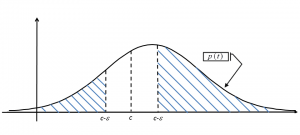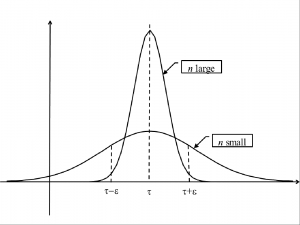19
Jan 17

## The law of large numbers proved### The law of large numbers overview

2. simulations in Excel show that convergence is not as fast as some textbooks claim;
3. to distinguish the law of large numbers from the central limit theorem read this;
4. the ultimate purpose is the application to simple regression with a stochastic regressor.

Here we busy ourselves with the proof.

### Measuring deviation of a random variable from a constant

Let$X$ be a random variable and$c$ some constant. We want a measure of$X$ differing from the constant by a given number$\varepsilon$ or more. The set where$X$ differs from$c$ by$\varepsilon>0$ or more is the outside of the segment$[c-\varepsilon,c+\varepsilon]$, that is,$\{|X-c|\ge\varepsilon\}=\{X\le c-\varepsilon\}\cup\{X\ge c+\varepsilon\}$.Figure 1. Measuring the outside of interval

Now suppose$X$ has a density$p(t)$. It is natural to measure the set$\{|X-c|\ge\varepsilon\}$ by the probability$P(|X-c|\ge\varepsilon)$. This is illustrated in Figure 1.

### Convergence to a spike formalizedFigure 2. Convergence to a spike

Once again, check out the idea. Consider a sequence of random variables$\{T_n\}$ and a parameter$\tau$. Fix some$\varepsilon>0$ and consider a corridor$[\tau-\varepsilon,\tau+\varepsilon]$ of width$2\varepsilon$ around$\tau$. For$\{T_n\}$ to converge to a spike at$\tau$ we want the area$P(|T_n-\tau|\ge\varepsilon)$ to go to zero as we move along the sequence to infinity. This is illustrated in Figure 2, where, say,$\{T_1\}$ has a flat density and the density of$\{T_{1000}\}$ is chisel-shaped. In the latter case the area$P(|T_n-\tau|\ge\varepsilon)$ is much smaller than in the former. The math of this phenomenon is such that$P(|T_n-\tau|\ge\varepsilon)$ should go to zero for any$\varepsilon>0$ (the narrower the corridor, the further to infinity we should move along the sequence).

Definition. Let$\tau$ be some parameter and let$\{T_n\}$ be a sequence of its estimators. We say that$\{T_n\}$ converges to$\tau$ in probability or, alternatively,$\{T_n\}$ consistently estimates$\tau$ if$P(|T_n-\tau|\ge\varepsilon)\rightarrow 0$ as$n\rightarrow 0$ for any$\varepsilon>0$.

### The law of large numbers in its simplest form

Let$\{X_n\}$ be an i.i.d. sample from a population with mean$\mu$ and variance$\sigma^2$. This is the situation from the standard Stats course. We need two facts about the sample mean$\bar{X}$: it is unbiased,

(1)$E\bar{X}=\mu$,

and its variance tends to zero

(2)$Var(\bar{X})=\sigma^2/n\rightarrow 0$ as$n\rightarrow 0$.

Now$P(|\bar{X}-\mu|\ge \varepsilon)$ (by (1))$=P(|\bar{X}-E\bar{X}|\ge \varepsilon)$ (by the Chebyshev inequality, see Extension 3))$\le\frac{1}{\varepsilon^2}Var(\bar{X})$ (by (2))$=\frac{\sigma^2}{n\varepsilon^2}\rightarrow 0$  as$n\rightarrow 0$.

Since this is true for any$\varepsilon>0$, the sample mean is a consistent estimator of the population mean. This proves Example 1.

### Final remarks

The above proof applies in the next more general situation.

Theorem. Let$\tau$ be some parameter and let$\{T_n\}$ be a sequence of its estimators such that: a)$ET_n=\tau$ for any$n$ and b)$Var(T_n)\rightarrow 0$. Then$\{T_n\}$ converges in probability to$\tau$.

This statement is often used on the Econometrics exams of the University of London.

In the unbiasedness definition the sample size is fixed. In the consistency definition it tends to infinity. The above theorem says that unbiasedness for all$n$ plus$Var(T_n)\rightarrow 0$ are sufficient for consistency.